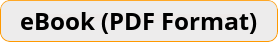\$13.99

# TASC Math Exercise Book A Comprehensive Workbook + TASC Math Practice TestsTASC Math test-takers #1 Choice! Recommended by Test Prep Experts!
TASC Math Exercise Bookwhich reflects the 2022 test guidelines and topics, provides students with the confidence and math skills they need to succeed on the TASC Math test. After completing this workbook, TASC Math test-takers will have a solid foundation and adequate practice that is necessary to ace the TASC Math test.Teacher's Choice
100% Guaranteed
Secure Checkout

TASC Math Exercise Book helps test takers learn how to solve TASC Math problems. Test takers learn how to build deep-rooted conceptual knowledge as well as practical fluency in basic mathematics. Through this math workbook, test takers learn how to work on actual TASC Math problems, improve their mathematics thinking, and produce solutions.

This updated version of the book offers a complete review of the TASC Math test, including:

• Arithmetic and Number Operations
• Algebra and Functions,
• Geometry and Measurement
• Data analysis, Statistics, & Probability